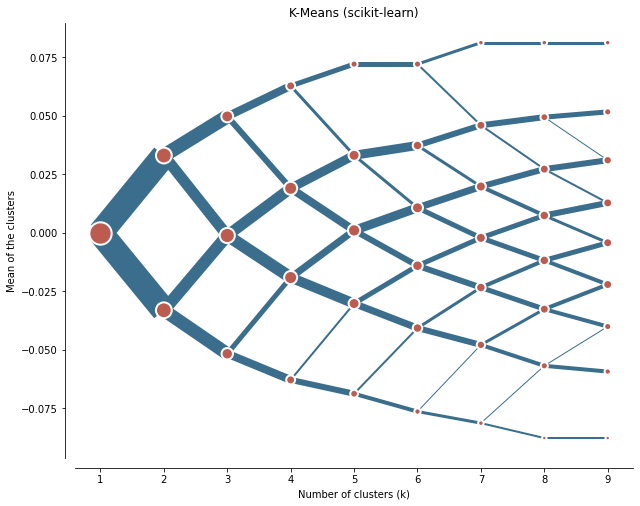Instantly share code, notes, and snippets.

# thistleknot/ecdf.py

Last active August 29, 2021 03:17
Star You must be signed in to star a gist
ECDF Python
This file contains bidirectional Unicode text that may be interpreted or compiled differently than what appears below. To review, open the file in an editor that reveals hidden Unicode characters. Learn more about bidirectional Unicode characters
 converted = pd.DataFrame() def ecdf2(values): co = len(values) externalArray = pd.DataFrame() for d in range(0,len(values.columns)): internalArray = [] for i in range(0,len(values.iloc[:,d])): a = ( \ sum( ( values.iloc[:,d] <= values.iloc[:,d][i] ) ) + \ sum( ( values.iloc[:,d] < values.iloc[:,d][i] ) ) \ ) / 2 / co internalArray.append(a) externalArray = pd.concat([externalArray,pd.DataFrame(internalArray).round(2)],axis=1) return(externalArray) converted = ecdf2(X_pca) converted

### thistleknot commented May 6, 2021 • edited

and you'll get something like thisI got an exact 50/50 split on my a 2 k cluster but subsequent clusters begin to split off into different non symmetrical configurations.

To get an even split on k means using the above "converted"

Before running the above code you need PCA converted variables

``````from sklearn.decomposition import PCA
from statsmodels.distributions.empirical_distribution import ECDF

pca = PCA(n_components=0.99, svd_solver='full')
pca.fit(scale(all_data.iloc[:,1:]))
#pca.explained_variance_
print(pca.explained_variance_ratio_.cumsum())

X_pca = pd.DataFrame(pca.transform(all_data.iloc[:,1:]))
ecdf = X_pca.apply(ECDF, axis=0)

X_pca.index = all_data.index
#X_pca.sort_values(by=,ascending=False,inplace=True)
#X_pca
``````

then run above code

then run below to apply a PCA variance scale

``````
import scipy.stats as st

#sum(1-pca.explained_variance_ratio_)
scaled_pca_var = (1-(1-pca.explained_variance_ratio_.cumsum()))/sum(1-(1-pca.explained_variance_ratio_.cumsum()))

pca_ecdf_scaled = pd.DataFrame()
for i in range(0,len(converted.columns)):
inner_scale = pd.DataFrame(st.norm.ppf(converted.iloc[:,i]))*scaled_pca_var[i]
#inner_scale = pd.DataFrame((ecdf[i].y)).mul(pca.explained_variance_ratio_[i],axis=0)
#plt.plot(pd.DataFrame(st.norm.ppf(ecdf[i].y)).mul(pca.explained_variance_ratio_[i],axis=0))
#plt.show()
pca_ecdf_scaled = pd.concat([pca_ecdf_scaled,inner_scale],axis=1)

pca_ecdf_scaled.index = all_data.index
``````

and finally feed through clustergram with pca_weighted=false (we just applied it)

``````from sklearn.preprocessing import scale

cgram = Clustergram(range(1, 10), n_init=1000)
#cgram.fit(scale(all_data.iloc[:,1:]))
cgram.fit(pca_ecdf_scaled)

ax = cgram.plot(
figsize=(10, 8),
line_style=dict(color=ugg.COLORS),
cluster_style={"color": ugg.COLORS},
pca_weighted=False
)
ax.yaxis.grid(False)
sns.despine(offset=10)
ax.set_title('K-Means (scikit-learn)')
``````